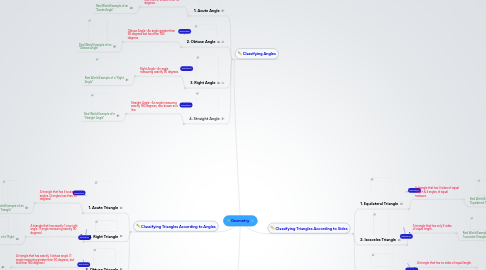# Geometry

Just an initial demo map, so that you don't start with an empty map list ...

Get Started. It's FreeGeometry## 1. Classifying Triangles According to Angles

### 1.1. 1. Acute Triangle

1.1.1. A triangle that has 3 acute angles. (3 angles less than 90 degrees)

1.1.1.1. Real World Example of an "Acute Triangle"

### 1.2. 2. Right Triangle

1.2.1. A triangle that has exactly 1 one right angle. (1 angle measuring exactly 90 degrees)

1.2.1.1. Real World Example of a "Right Triangle"

### 1.3. 3. Obtuse Triangle

1.3.1. A triangle that has exactly 1 obtuse angle. (1 angle measuring greater than 90 degrees, but less than 180 degrees)

1.3.1.1. Real World Example of an "Obtuse Triangle"

## 2. Classifying Angles

### 2.1. 1. Acute Angle

2.1.1. Acute Angle--An angle that is less than or smaller than 90 degrees.

2.1.1.1. Real World Example of an "Acute Angle"

### 2.2. 2. Obtuse Angle

2.2.1. Obtuse Angle--An angle greater than 90 degrees but less than 180 degrees.

2.2.1.1. Real World Example of an "Obtuse Angle"

### 2.3. 3. Right Angle

2.3.1. Right Angle--An angle measuring exactly 90 degrees.

2.3.1.1. Real World Example of a "Right Angle"

### 2.4. 4. Straight Angle

2.4.1. Straight Angle--An angle measuring exactly 180 degrees, also known as a line.

2.4.1.1. Real World Example of a "Straight Angle"

## 3. Classifying Triangles According to Sides

### 3.1. 1. Equilateral Triangle

3.1.1. A triangle that has 3 sides of equal length & 3 angles of equal measure.

3.1.1.1. Real World Example of an "Equilateral Triangle"

### 3.2. 2. Isosceles Triangle

3.2.1. A triangle that has only 2 sides of equal length.

3.2.1.1. Real World Example of an "Isosceles Triangle"

### 3.3. 3. Scalene Triangle

3.3.1. A triangle that has no sides of equal length.

3.3.1.1. Real World Example of a "Scalene Triangle"